# 0 前言

我想认真写好快速傅里叶变换（Fast Fourier Transform，FFT），所以这篇文章会由浅到细，由窄到宽的讲解，但是傅里叶变换对于寻常人并不是很容易理解的，所以对于基础不牢的人我会通过前言普及一下相关知识。

我们复习一下三角函数的标准式：

$$y=A\cos (\omega x+\theta )+k$$

$A$代表振幅，函数周期是$\frac{2\pi}{w}$，频率是周期的倒数$\frac{w}{2\pi}$，$\theta$是函数初相位，$k$在信号处理中称为直流分量。这个信号在频域就是一条竖线。

我们再来假设有一个比较复杂的时域函数$y=f(t)$，根据傅里叶的理论，任何一个周期函数可以被分解为一系列振幅A，频率$\omega$或初相位$\theta$正弦函数的叠加

$$y = A_1sin(\omega_1t+\theta_1) + A_2sin(\omega_2t+\theta_2) + A_3sin(\omega_3t+\theta_3)$$

该信号在频域有三条竖线组成，而竖线图我们把它称为频谱图，大家可以通过下面的动画了解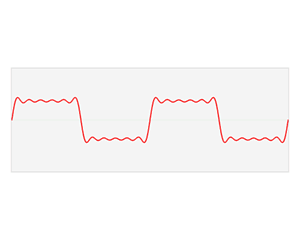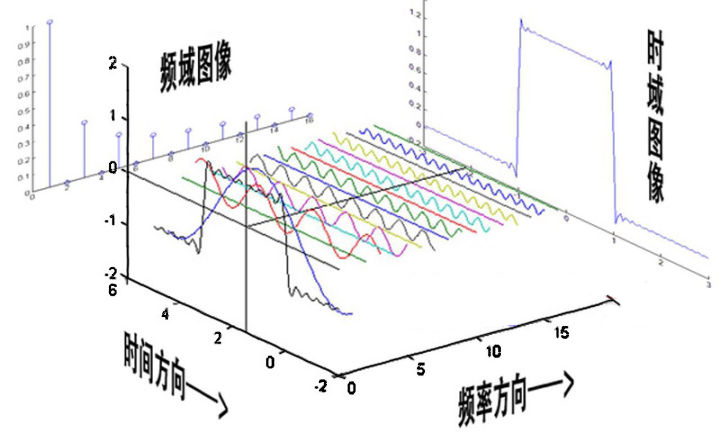如图可知，通过时域到频域的变换，我们得到了一个从侧面看的频谱，但是这个频谱并没有包含时域中全部的信息。因为频谱只代表每一个对应的正弦波的振幅是多少，而没有提到相位。基础的正弦波$Asin(wt+\theta )$中，振幅，频率，相位缺一不可不同相位决定了波的位置，所以对于频域分析，仅仅有频谱（振幅谱）是不够的，我们还需要一个相位谱。

我依稀记得高中学正弦函数的是时候，$\theta$的多少决定了正弦波向右移动多少。当然那个时候横坐标是相位角度，而时域信号的横坐标是时间，因此我们只需要将时间转换为相位角度就得到了初相位。相位差则是时间差在一个周期中所占的比例

$$2\pi \frac{t}{T}$$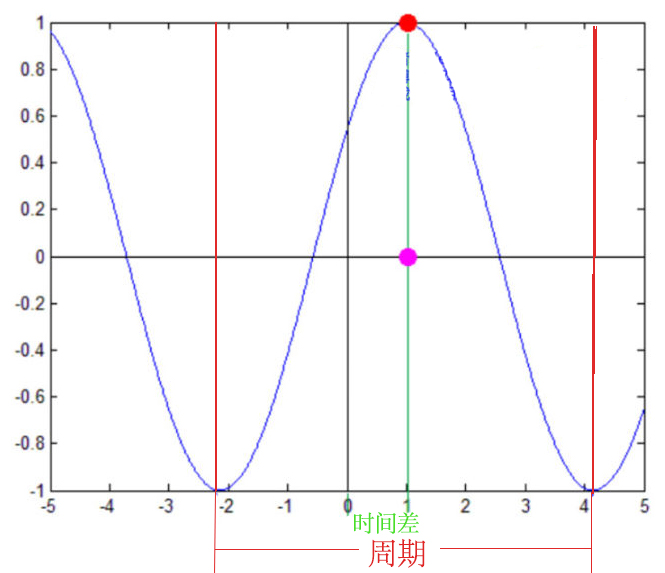所以傅里叶变换可以把一个比较复杂的函数转换为多个简单函数的叠加，将时域（即时间域）上的信号转变为频域（即频率域）上的信号，看问题的角度也从时间域转到了频率域，因此在时域中某些不好处理的地方，在频域就可以较为简单的处理，这就可以大量减少处理信号计算量。信号经过傅里叶变换后，可以得到频域的幅度谱以及相位谱，信号的相位谱幅度谱是信号傅里叶变换后频谱的两个属性

• 时域复杂的函数，在频域就是几条竖线
• 求解微分方程，傅里叶变换则可以让微分和积分在频域中变为乘法和除法

## 傅里叶变换相关函数

假设我们的输入信号的函数是

$$S=0.2+0.7*\cos (2\pi*50t+\frac{20}{180}\pi)+0.2*\cos (2\pi*100t+\frac{70}{180}\pi)$$

freqs = np.fft.fftfreq(采样数量, 采样周期)　　通过采样数与采样周期得到时域序列经过傅里叶变换后的频率序列

np.fft.fft(原序列)　　原函数值的序列经过快速傅里叶变换得到一个复数数组，复数的模代表的是振幅，复数的辐角代表初相位

np.fft.ifft(复数序列)　　复数数组 经过逆向傅里叶变换得到合成的函数值数组import matplotlib.pyplot as plt
import numpy as np
import numpy.fft as fft

plt.rcParams['font.sans-serif']=['SimHei'] #用来正常显示中文标签
plt.rcParams['axes.unicode_minus']=False #用来正常显示符号

Fs = 1000;            # 采样频率
T = 1/Fs;             # 采样周期
L = 1000;             # 信号长度
t = [i*T for i in range(L)]
t = np.array(t)

S = 0.2+0.7*np.cos(2*np.pi*50*t+20/180*np.pi) + 0.2*np.cos(2*np.pi*100*t+70/180*np.pi) ;

complex_array = fft.fft(S)
print(complex_array.shape)  # (1000,)
print(complex_array.dtype)  # complex128
print(complex_array)  # (-2.360174309695419e-14+2.3825789764340993e-13j)

#################################
plt.subplot(311)
plt.grid(linestyle=':')
plt.plot(1000*t[1:51], S[1:51], label='S')  # y是1000个相加后的正弦序列
plt.xlabel("t（毫秒）")
plt.ylabel("S(t)幅值")
plt.title("叠加信号图")
plt.legend()

###################################
plt.subplot(312)
S_ifft = fft.ifft(complex_array)
# S_new是ifft变换后的序列
plt.plot(1000*t[1:51], S_ifft[1:51], label='S_ifft', color='orangered')
plt.xlabel("t（毫秒）")
plt.ylabel("S_ifft(t)幅值")
plt.title("ifft变换图")
plt.grid(linestyle=':')
plt.legend()

###################################
# 得到分解波的频率序列
freqs = fft.fftfreq(t.size, t - t)
# 复数的模为信号的振幅（能量大小）
pows = np.abs(complex_array)

plt.subplot(313)
plt.title('FFT变换,频谱图')
plt.xlabel('Frequency 频率')
plt.ylabel('Power 功率')
plt.tick_params(labelsize=10)
plt.grid(linestyle=':')
plt.plot(freqs[freqs > 0], pows[freqs > 0], c='orangered', label='Frequency')
plt.legend()
plt.tight_layout()
plt.show()
python代码实现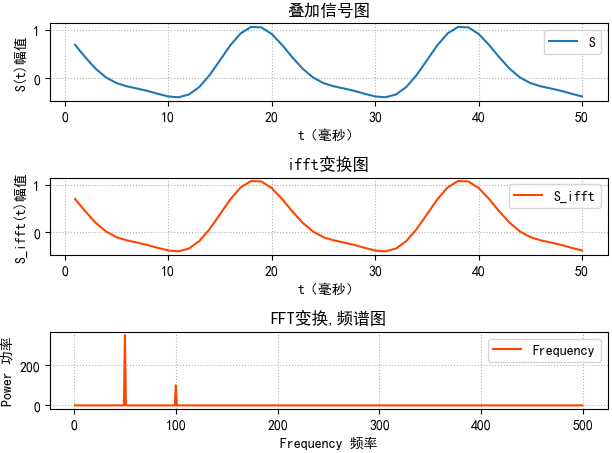clear
clc
close all
Fs = 1000;            % Sampling frequency
T = 1/Fs;             % Sampling period
L = 1000;             % Length of signal
t = (0:L-1)*T;        % Time vector
S = 0.2-0.7*cos(2*pi*50*t+20/180*pi) + 0.2*cos(2*pi*100*t+70/180*pi) ;
plot(1000*t(1:50),S(1:50))
title('叠加信号图')
xlabel('t (milliseconds)')
ylabel('S(t)')

figure
Y = fft(S);
P2 = abs(Y/L);
P1 = P2(1:L/2+1);
P1(2:end-1) = 2*P1(2:end-1);
f = Fs*(0:(L/2))/L;
plot(f,P1,'linewidth',2)
title('FFT变换')
xlabel('频率(Hz)')
ylabel('幅值')

figure
pred_X=ifft(Y);
plot(1000*t(1:50),pred_X(1:50),'r-')
MATLAB实现

## 基于傅里叶变换的频域滤波

1、读取音频文件，获取音频文件基本信息：采样个数，采样周期，与每个采样的声音信号值。绘制音频时域的：时间/位移图像

import numpy as np
import numpy.fft as nf
import scipy.io.wavfile as wf
import matplotlib.pyplot as plt

# 读取音频文件
print(sample_rate)  # sample_rate：采样率44100
print(noised_sigs.shape)    # noised_sigs:存储音频中每个采样点的采样位移(220500,)
times = np.arange(noised_sigs.size) / sample_rate

plt.figure('Filter')
plt.subplot(221)
plt.title('Time Domain', fontsize=16)
plt.ylabel('Signal', fontsize=12)
plt.tick_params(labelsize=10)
plt.grid(linestyle=':')
plt.plot(times[:178], noised_sigs[:178], c='orangered', label='Noised')
plt.legend()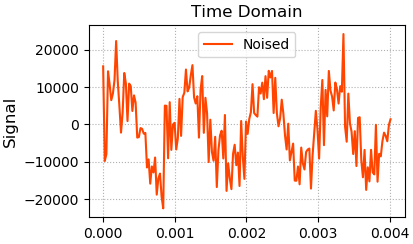2、基于傅里叶变换，获取音频频域信息，绘制音频频域的：频率/能量图像

# 傅里叶变换后，绘制频域图像
freqs = nf.fftfreq(times.size, times - times)
complex_array = nf.fft(noised_sigs)
pows = np.abs(complex_array)

plt.subplot(222)
plt.title('Frequency Domain', fontsize=16)
plt.ylabel('Power', fontsize=12)
plt.tick_params(labelsize=10)
plt.grid(linestyle=':')
# 指数增长坐标画图
plt.semilogy(freqs[freqs > 0], pows[freqs > 0], c='limegreen', label='Noised')
plt.legend()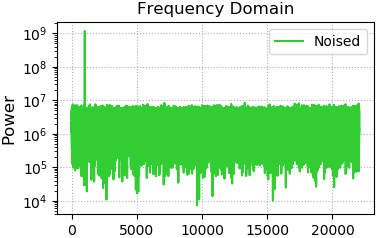3、将低频噪声去除后绘制音频频域的：频率/能量图像

# 寻找能量最大的频率值
fund_freq = freqs[pows.argmax()]
# where函数寻找那些需要抹掉的复数的索引
noised_indices = np.where(freqs != fund_freq)
# 复制一个复数数组的副本，避免污染原始数据
filter_complex_array = complex_array.copy()
filter_complex_array[noised_indices] = 0
filter_pows = np.abs(filter_complex_array)

plt.subplot(224)
plt.xlabel('Frequency', fontsize=12)
plt.ylabel('Power', fontsize=12)
plt.tick_params(labelsize=10)
plt.grid(linestyle=':')
plt.plot(freqs[freqs >= 0], filter_pows[freqs >= 0], c='dodgerblue', label='Filter')
plt.legend()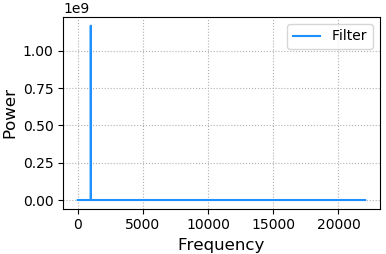4、基于逆向傅里叶变换，生成新的音频信号，绘制音频时域的：时间/位移图像

filter_sigs = nf.ifft(filter_complex_array).real
plt.subplot(223)
plt.xlabel('Time', fontsize=12)
plt.ylabel('Signal', fontsize=12)
plt.tick_params(labelsize=10)
plt.grid(linestyle=':')
plt.plot(times[:178], filter_sigs[:178], c='hotpink', label='Filter')
plt.legend()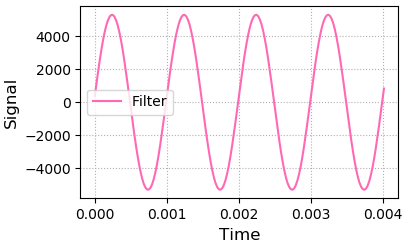5、重新生成音频文件

# 生成音频文件
wf.write('./da_data/filter.wav', sample_rate, filter_sigs)
plt.show()

## 离散傅里叶变换（DFT）

离散傅里叶变换（DFT）对有限长时域离散信号的频谱进行等间隔采样，频域函数被离散化了，便于信号的计算机处理。DFT的运算量太大，FFT是离散傅里叶变换的快速算法。

说白了FFT和DFT它俩就是一个东东，只不过复杂度不同，

有时候我们能够看到N点傅里叶变换，我个人理解是这个N点是信号前面N个连续的数值，即N点FFT意思就是截取前面N个信号进行FFT，这样就要求我们的前N个采样点必须包含当前信号的一个周期，不然提取的余弦波参数与正确的叠加波的参数相差很大。

如果在N点FFT的时候，如果这N个采样点不包含一个周期呢？或者说我们的信号压根不是一个周期函数咋办？或者有一段是噪音数据呢？如果用FFT计算，就会对整体结果影响很大，然后就有人想通过局部来逼近整体，跟微积分的思想很像，将信号分成一小段一小段，然后对每一小段做FFT，就跟分段函数似的，无数个分段函数能逼近任意的曲线((⊙o⊙)…应该没错吧)，这样每一段都不会互相影响到了。

# 短时傅里叶变换stft

在短时傅里叶变换过程中，窗的长度决定频谱图的时间分辨率和频率分辨率，窗长越长，截取的信号越长，信号越长，傅里叶变换后频率分辨率越高，时间分辨率越差；相反，窗长越短，截取的信号就越短，频率分辨率越差，时间分辨率越好，也就是说短时傅里叶变换中，时间分辨率和频率分辨率之间不能兼得，应该根据具体需求进行取舍。

• 每个窗口的长度：nsc
• 每相邻两个窗口的重叠率：nov
• 每个窗口的FFT采样点数：nff

• 海明窗：w=hamming(nsc, 'periodic')
• 信号被分成了多少片
• 短时傅里叶变换：

python库librosa实现

librosa.stft(y, n_fft=2048, hop_length=None, win_length=None, 　　　　　　　　window='hann', center=True, pad_mode='reflect')

• 复数的实部：np.abs(D(f,t))频率的振幅
• 复数的虚部：np.angle(D(f,t))频率的相位

• y：音频时间序列
• n_fftFFT窗口大小，n_fft=hop_length+overlapping
• hop_length帧移，如果未指定，则默认win_length / 4。
• win_length每一帧音频都由window（）加窗。窗长win_length，然后用零填充以匹配N_FFT。默认win_length=n_fft
• window：字符串，元组，数字，函数 shape =（n_fft, )
• 窗口（字符串，元组或数字）；
• 窗函数，例如scipy.signal.hanning
• 长度为n_fft的向量或数组
• center：bool
• 如果为True，则填充信号y，以使帧 D [:, t]以y [t * hop_length]为中心。
• 如果为False，则D [:, t]从y [t * hop_length]开始
• dtypeD的复数值类型。默认值为64-bit complex复数

• STFT矩阵，shape =（1 + $\frac{n_{fft} }{2}$，t）

tensorflow实现

# [batch_size, signal_length].  batch_size and signal_length 可能会不知道
signals = tf.placeholder(tf.float32, [None, None])

# stfts 短时傅里叶变换：就是对信号中每一帧信号进行傅里叶变换
# shape is [batch_size, ?, fft_unique_bins]
# 其中 fft_unique_bins = fft_length // 2 + 1 = 513.
stfts = tf.contrib.signal.stft(signals, frame_length=1024, frame_step=512,
fft_length=1024)

wlen：窗长

frame_length是信号中帧的长度

frame_step是帧移

fft_length：做fft变换的长度，或一种说话：fft变换所取得N点数，在有些地方也表示为NFFT。

FFT后的分辨率（频率的间隔）为fs/NFFT。当NFFT>wlen时就是在数据补零后做FFT，做的FFT得到的频谱等于以wlen长数据FFT的频谱中内插。

numpy库实现def wav_to_frame(wave_data, win_len, win_shift):
"""
进行分帧操作
:param wave_data: 原始的数据
:param win_len: 滑动窗长
:param win_shift: 滑动间隔
:return: 分帧之后的结果，输出一个帧矩阵
"""
num_frames = (len(wave_data) - win_len) // win_shift + 1
results = []
for i in range(num_frames):
results.append(wave_data[i*win_shift:i*win_shift + win_len])
return np.array(results)

def spectrum_power(frames, NFFT):
"""
计算每一帧傅立叶变换以后的功率谱
参数说明：
frames:audio2frame函数计算出来的帧矩阵
NFFT:FFT的大小
"""
# 功率谱等于每一点的幅度平方/NFFT
return 1.0/NFFT * np.square(spectrum_magnitude(frames, NFFT))

def spectrum_magnitude(frames, NFFT):
"""计算每一帧经过FFT变幻以后的频谱的幅度，若frames的大小为N*L,则返回矩阵的大小为N*NFFT
参数：
frames:即audio2frame函数中的返回值矩阵，帧矩阵
NFFT:FFT变换的数组大小,如果帧长度小于NFFT，则帧的其余部分用0填充铺满
"""
complex_spectrum = np.fft.rfft(frames, NFFT)    # 对frames进行FFT变换
# 返回频谱的幅度值
return np.absolute(complex_spectrum)
View Code

# frequency bin

raw data 经过FFT后得到的频谱图中，频率轴的频率间隔或分辨率，通常取决采样速率和采样点数据。
功率谱中的频率点或线的数量为$\frac{N_{recode}}{2}$  ，$N_{recode}$  是信号在时域的采样点数。
功率谱的第一个频点始终为直流（频率=0），最后一个频点为$\frac{f_{sample}}{2}-\frac{f_{sample}}{N_{recode}}$  。频点采用相等的间隔$\frac{f_{sample}}{N_{recode}}$  ，通常用频率窗口或FFT窗口表示。窗口（Bin）可以由数据转换器的采样周期计算：
$$bin=采样率/采样点数=\frac{f_{sample}}{N_{recode}}=\frac{1}{N_{recode}*\Delta t_{sample}}$$

# 参考

【音频处理】离散傅里叶变换

【音频处理】短时傅里叶变换

posted @ 2019-09-20 19:18  凌逆战  阅读(...)  评论(...编辑  收藏